Related Articles

# Indefinite Integrals

• Last Updated : 16 Jun, 2021

The derivatives have been really useful in almost every aspect of life. They allow to find the rate of change of a function. Sometimes there are situations where the derivative of a function is available and the goal is to calculate the actual function whose derivative is given. In these cases, integrals come into play. Intuitively they are the reverse of the differentiation process. Integrals also have a lot of applications in calculus as well as real life. They are useful in analyzing functions, calculating the area and volumes of different arbitrary shapes.

### Introduction to Integrals

Integrals are also known as anti-derivatives. Integration is the inverse process of differentiation. Instead of differentiating a function, we are given the derivative of a function and are required to calculate the function from the derivative. This process is called integration or anti-differentiation. Consider a function f(x) = sin(x), the derivative of this function if f'(x) = cos(x). So, the integration of f'(x) should give back the function f(x). Notice that for every function f(x) = sin (x) + C, the derivative is same because constant becomes zero after differentiation. Thus, anti-derivatives are not unique, for every function, its anti-derivatives are infinite.This constant C is called arbitrary constant.

A new symbol is used for denoting integrals. This will represent the integration operation over any function. The table below represents the symbols and meanings related to integrals.

There are certain formulas and rules which when kept in mind, help us simplify the calculating and do it fast. The reverse power rule is one of the rules which help us in the integration of polynomials and other functions.

Reverse Power Rule

This rule helps in integrating the functions which have terms of the form xnHere, C is the arbitrary constant and n ≠ 1.

In this rule, the exponent of the variable is increased by 1 and then the result is divided by the new exponent value. The table below gives integrals of some standard functions.

Rewriting the Integrals

Sometimes when functions become too complex, it becomes hard to integrate them. There are certain properties of integrals that help in simplifying and rewriting the integrals.

Property 1:Property 2:Property 3:### Visual Interpretation of Integrals

Apart from the usual algebraic rules of calculating the integrals. Integrals can be understood through graphs. It is clear that integrals are nothing but anti-derivatives. Consider a function f(x), and let’s say it’s anti-derivative if given by F(x). In that case, F'(x) = f(x). Consider the graph below as the graph of the function f(x), it means that the graph of the derivatives of the function F(x) is given and the goal is to determine the integral function F(x).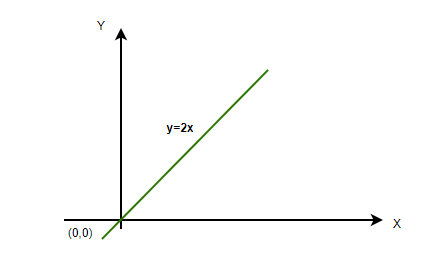The graph shows the function f(x) = 2x, it is a straight line passing through the origin. Let’s integrate the given function using the reverse power rule mentioned above.⇒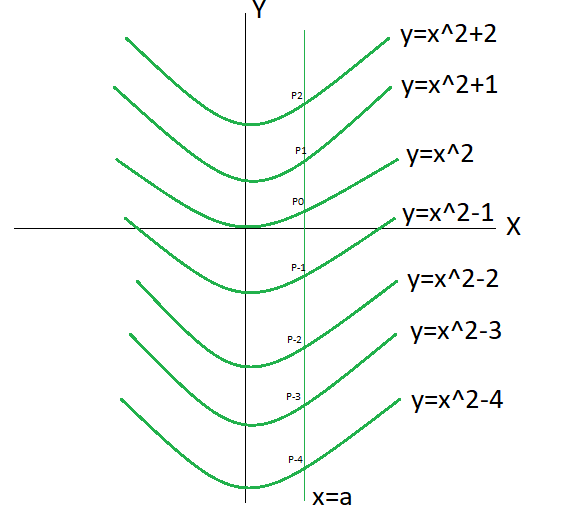Now, when this C = 0, the equation of the integral becomes F(x) = x2 , which is a parabola centered at the origin. When C =1, the parabola is shifted upwards by one unit and similarly at C = -1, the parabola is shifted downwards by one unit.

This means that function F(x) = x2 + C represents a family of curves.

Determining Integrals by Graphs

Integrals can be determined roughly by the graphs. Integrands are nothing but the derivatives of the integrals. They give information about the rate of increase/decrease and maxima and minima of the integrals. Let us consider a graph of a function f(x),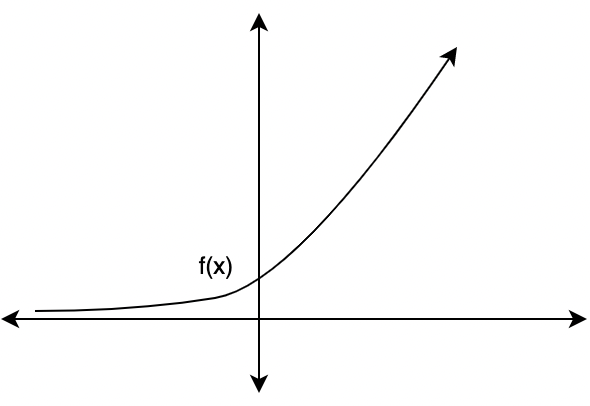Assuming, F(x) =Since the derivative of the function is positive and increasing, the function will increase at an increasing rate, the graph of the function F(x) will approximately look like a parabola that is rising upwards. The figure below gives a rough idea of the graph of the function F(x).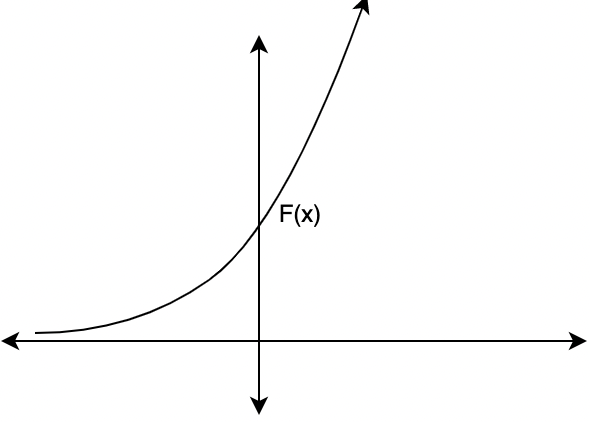Let’s see some sample problems

### Sample Problems

Question 1: Find the integral for the given function f(x),

f(x) = sin(x) + 1

Solution:

Given f(x) = sin(x) + 1

sin(x) is a standard function, and it’s anti-derivative is known.Using the property 2 mentioned above,Question 2: Find the integral for the given function f(x),

f(x) = 2ex

Solution:

Given f(x) = 2ex

ex is a standard function, and it’s anti-derivative is known.Using the property 1 mentioned above,⇒2ex + C

Question 3: Find the integral for the given function f(x),

f(x) = 5x-2

Solution:

Given f(x) = 5x-2

Using the reverse power ruleUsing the property 1 mentioned above,Question 4: Find the integral for the given function f(x),

f(x) = sin(x) + 5cos(x)

Solution:

Given f(x) = sin(x) + 5cos(x)

sin(x) and cos(x) are standard functions, and its integral is known.Using the properties 1 and 2 mentioned above,Question 5: Find the integral for the given function f(x),

f(x) = 5x-2 + x4 + x

Solution:

Given f(x) = 5x-2 + x4 + x

Using the reverse power ruleUsing the properties 1 and 2 and power rule mentioned above,Question 6: For the function graph given below, draw a rough graph of the integral.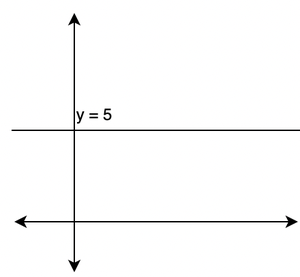Solution:

The given graph is constant, that y = 5.

Since this graph is the graph of the derivative of its integral. This means the graph of the integral is constantly increasing. It must be a straight will positive slope.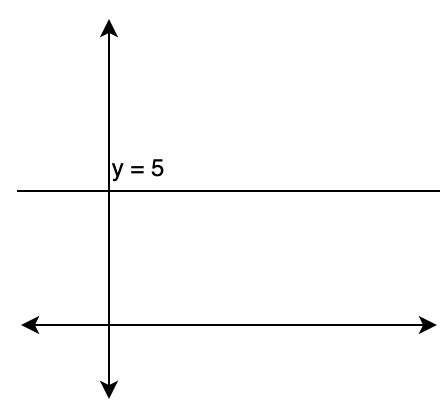Attention reader! Don’t stop learning now. Join the First-Step-to-DSA Course for Class 9 to 12 students , specifically designed to introduce data structures and algorithms to the class 9 to 12 students

My Personal Notes arrow_drop_up